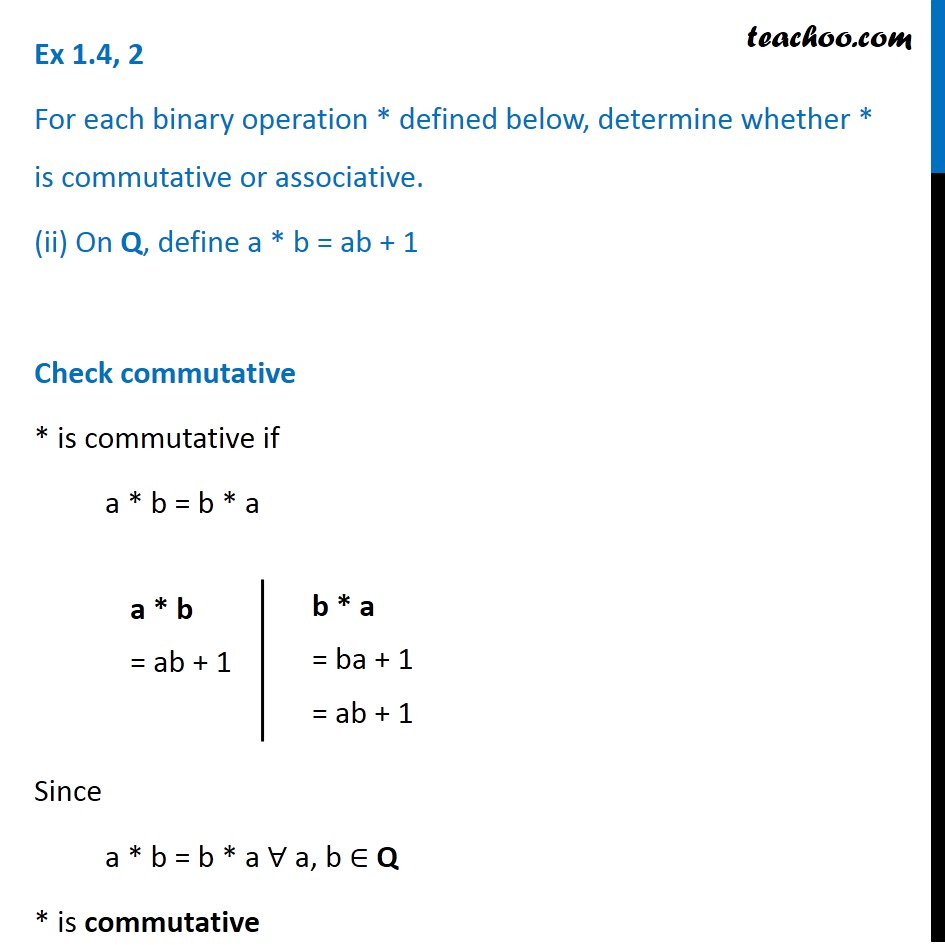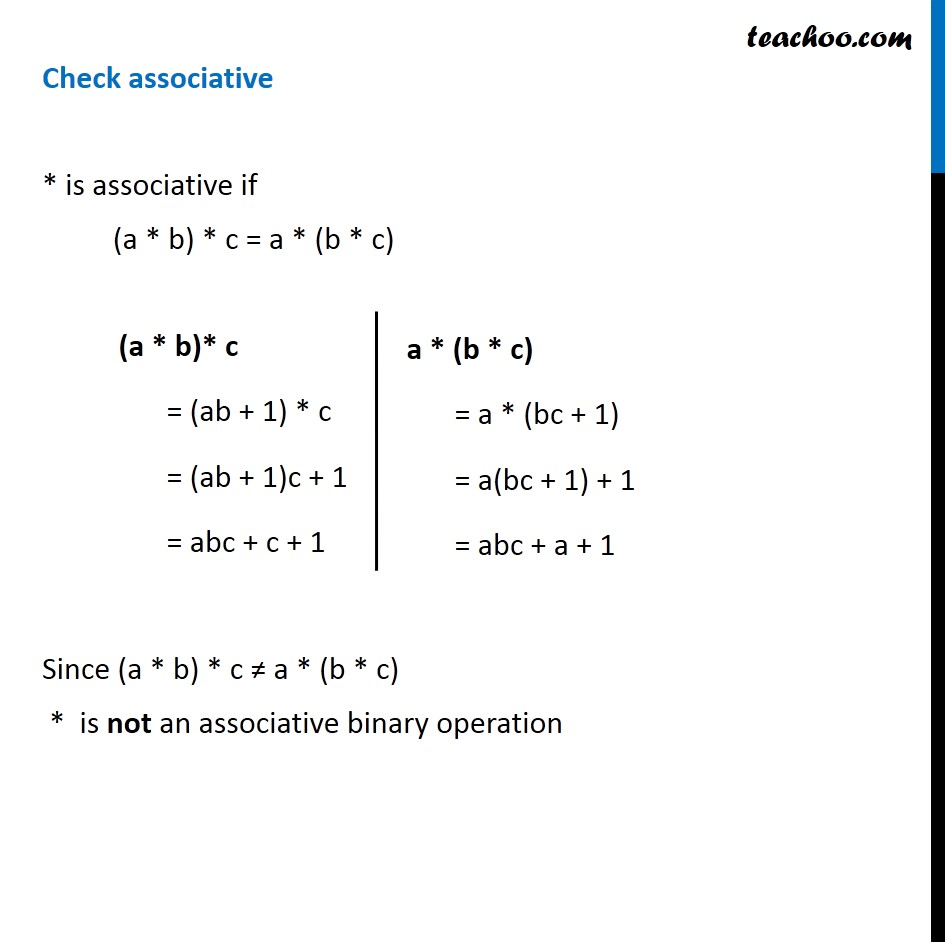Ex 1.4

Chapter 1 Class 12 Relation and Functions
Serial order wiseIntroducing your new favourite teacher - Teachoo Black, at only ₹83 per month

### Transcript

Ex 1.4, 2 For each binary operation * defined below, determine whether * is commutative or associative. (ii) On Q, define a * b = ab + 1 Check commutative * is commutative if a * b = b * a Since a * b = b * a ∀ a, b ∈ Q * is commutative a * b = ab + 1 b * a = ba + 1 = ab + 1 Check associative * is associative if (a * b) * c = a * (b * c) Since (a * b) * c ≠ a * (b * c) * is not an associative binary operation (a * b)* c = (ab + 1) * c = (ab + 1)c + 1 = abc + c + 1 a * (b * c) = a * (bc + 1) = a(bc + 1) + 1 = abc + a + 1 a * (b * c) = a * (bc + 1) = a(bc + 1) + 1 = abc + a + 1